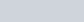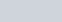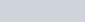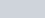Draw a right triangle ABC in which AC = AB = 4.5 cm and ∠A = 90°.
Question:

Draw a right triangle ABC in which AC = AB = 4.5 cm and ∠A = 90°. Draw a triangle similar to ΔABC with its sides equal to (5/4)th of the corresponding sides of ΔABC.

Solution:

Given that

Construct a right triangle of sides $A B=A C=4.5 \mathrm{~cm}$, and $\angle A=90^{\circ}$ and then a triangle similar to it whose sides are $(5 / 4)^{\text {th }}$ of the corresponding sides of $\triangle A B C$.

We follow the following steps to construct the givenStep of construction

Step: I- First of all we draw a line segment.

Step: II- With as centre and draw an angle.

Step: III- With as centre and radius.

Step: IV- Join BC to obtain.

Step: V- Below AB, makes an acute angle.

Step: VI- Along $A X$, mark off five points $A_{1}, A_{2}, A_{3}, \mathrm{~A}_{4}$ and $\mathrm{A}_{5}$ such that $A A_{1}=A_{1} A_{2}=A_{2} A_{3}=A_{3} A_{4}=A_{4} A_{5}$’

Step: VII-Join $A_{4} B$.

Step: VIII- Since we have to construct a triangle each of whose sides is $(5 / 4)^{\text {th }}$ of the corresponding sides of $\triangle A B C$.

So, we draw a line $A_{5} B^{\prime}$ on $A X$ from point $A_{5}$ which is $A_{5} B^{\prime} \| A_{4} B$, and meeting $A B$ at $B$ ‘.

Step: IX- From B’ point draw $B^{\prime} C^{\prime} \| B C$, and meeting $A C$ at $C^{\prime}$

Thus, $\triangle A B^{\prime} C$ ‘ is the required triangle, each of whose sides is $(5 / 4)$ the corresponding sides of $\triangle A B C$.